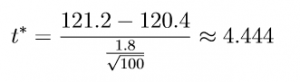# Hypothesis Testing for One – Sample Mean

So the t* test statistic is:## 4) Calculate the p-value

To determine the p-value, in addition to the t* test statistic above we also need to calculate the degrees of freedom. For our example, this is just the batch size subtracted by 1: 100 – 1 = 99.

The t* value 4.444 and the 99 degrees of freedom can be input into any statistical software or freely available web application to estimate the corresponding p-value of .000012.

## 5) Decide on the null hypothesis

As our p-value of 0.000012 is much lower than the significance level of 5% (aka 0.05) we reject the null hypothesis that company C’s CO2 mean is equal to the average value from different manufacturers.

## 6) State the overall conclusion

From the data given, at the significance level of 5% or the confidence level of 95%, we reject the null hypothesis in favor of the alternative, that company C’s cars’ CO2 emission is greater than the average value from different manufacturers.

# Hiring Data Scientist / Engineer

We are looking for Data Scientist and Engineer.